# Minimum Sum Path in a Matrix

## Introduction

In this article, we are going to discuss the Minimum Sum Path in a Matrix problem. Such problems are essential for learning as these questions help in building the foundation for good programmers. If someone is thorough with such straightforward questions, they are bound to have a promising future as a coder.

## Problem Statement

Given a cost matrix costMat[][] and a destination coordinate (i,j), we have to come up with a solution that returns the minimum sum path from (0,0) to (i,j). The condition of traversal is that we can travel only towards the right direction, below and diagonally below. The minimum sum path is the sum of all the costs of the path, including the source and destination coordinates.

## Approach 1(Recursion)

This approach is the most naive solution wherein we calculate the traversal cost in the three possible directions and return the minimum of the three. We use recursion on the calcMincost() function to do so. We first travel in all the three possible directions one by one and find the minimum. After adding the initial and final path cost to the calculated minimum path cost, we return the final answer.

The major drawback of this approach is that its computation time, in terms of time and space complexity, is the highest among the three techniques shown. It is because of recursion that we get overlapping solutions. Overlapping solution means that some of the computations are done multiple times, increasing the redundancy. Even though we have calculated a solution once, we do not store it; we calculate it again and again.

### Solution

The destination co-ordinates are stored in dest_i and dest_j. The three possible directions in which we can travel are - right, down, and diagonally lower. So from any given cell (i,j) we can travel to (i,j+1), (i+1, j), (i+1, j+1). We recursively call the calcMinCost() on these three paths, find the minimum of the three, and add the starting cell cost. The three recursive statements are -

calcMinCost(costMat, dest_i - 1, dest_j)

calcMinCost(costMat, dest_i, dest_j - 1)

calcMinCost(costMat, dest_i - 1, dest_j - 1)

For finding the minimum of the three, we have a user-defined function called min(), which takes three arguments and returns the minimum of the three.

#### C++ Implementation

``````#include <iostream>
#include <climits>

#define R 5
#define C 5
using namespace std;

int min(int x, int y, int z) {
if (x < y)
return (x < z) ? x : z;
else
return (y < z) ? y : z;
}

int calcMinCost(int costMat[R][C], int dest_i, int dest_j) {
if (dest_j < 0 || dest_i < 0)
return INT_MAX;
else if (dest_i == 0 && dest_j == 0)
return costMat[dest_i][dest_j];
else
return costMat[dest_i][dest_j] + min(calcMinCost(costMat, dest_i - 1, dest_j - 1), calcMinCost(costMat, dest_i - 1, dest_j), calcMinCost(costMat, dest_i, dest_j - 1));
}

int main()
{
int costMat[R][C] = { {1, 2, 3, 1, 2},
{4, 9, 2, 5, 6},
{3, 2, 3, 1, 9},
{2, 1, 3, 2, 7},
{1, 2, 5, 3, 1} };

cout << "The minimum sum path of the given matrix is: " << calcMinCost(costMat, 4, 4) << endl;

return 0;
}``````

#### Output: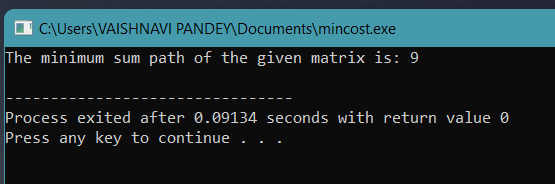#### Java Implementation

``````// Java Implementation
public class MinimumSumPath {

// To get the minimum of 3 integers..
static int min(int x, int y, int z)
{
if (x < y)
return (x < z) ? x : z;
else
return (y < z) ? y : z;
}

//Function to calculate the minimum cost between (0,0) and (a,b)
static int CalculateMinCost(int costMatrix[][],int a, int b)
{
if (a < 0 || b < 0)
return Integer.MAX_VALUE;
else if (a == 0 && b == 0)
return costMatrix[a][b];
else
return costMatrix[a][b] +
min( CalculateMinCost(costMatrix, a-1, b-1),
CalculateMinCost(costMatrix, a-1, b),
CalculateMinCost(costMatrix, a, b-1) );
}

// Driver code
public static void main(String args[])
{

int costMatrix[][] = {   {1, 2, 3, 1, 2},
{4, 9, 2, 5, 6},
{3, 2, 3, 1, 9},
{2, 1, 3, 2, 7},
{1, 2, 5, 3, 1} };

System.out.print("The minimum sum path of the given matrix is: " + CalculateMinCost(costMatrix, 4, 4));
}
}``````

#### Output: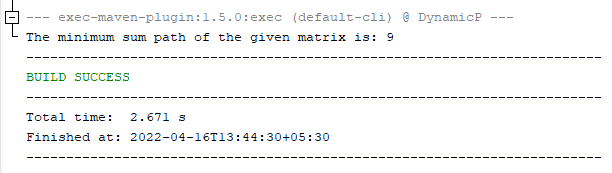#### Python Implementation

``````import sys
def calcMinCost( costMat, dest_i, dest_j):
if dest_j < 0 or dest_i < 0:
return sys.maxsize
elif dest_i == 0 and dest_j == 0:
return costMat[dest_i][dest_j]
else:
return costMat[dest_i][dest_j] + min(calcMinCost(costMat, dest_i - 1, dest_j - 1), calcMinCost(costMat, dest_i - 1, dest_j), calcMinCost(costMat, dest_i, dest_j - 1))

def main():
costMat = [ [1, 2, 3, 1, 2], [4, 9, 2, 5, 6], [3, 2, 3, 1, 9 ],[2, 1, 3, 2, 7], [1, 2, 5, 3, 1] ]

res = calcMinCost(costMat, 4, 4)
print("The minimum sum path of the given matrix is: ", res)

main()``````

#### Output: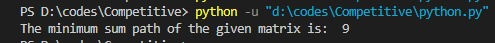## Time and Space Complexity

• Time Complexity
In this approach, we use recursion on the given matrix. For recursion, we call the calcMinCost() function thrice and pass the three possible directions of traversal. Due to this, the solution of Approach 1 is the worst (exponential) as compared to the other two approaches mentioned in this article. Thus, the time complexity is:

T(n) = 3^N,

Where N is the max of R and C.

• Space Complexity
In this approach, the recursive calling takes space in our memory. This memory is also known as ‘Recursive Stack Space.’ It is the mandatory space that will be occupied to implement the recursion. Thus,

Space Complexity = O(N)

## Approach 2(Dynamic Programming)

As we saw in Approach 1 that numerous calculations are done multiple times. This increases the redundancy of our code and affects the time complexity of our code exponentially. To overcome the redundant calculations and improve the time complexity of the code, we use dynamic programming.

Readers who are not familiar with the concepts of DP may first check out this blog: Dynamic Programming & algorithms

To apply dynamic programming to our problem, we first create a dynamic table dp[][] whose dimensions are similar to the given matrix. We set the first cell of DP the same as the first cell of the given matrix, that is, dp = costMat. Then starting from (0,0), we traverse to each cell and calculate the path cost. So, at any given instance, the minimum path cost of travelling from (0,0) to that cell is equal to the cell value.

The code solution for this approach is given below.

#### C++ Implementation

``````#include <iostream>
#define R 5
#define C 5
using namespace std;

void printCostMatrix(int costMat[R][C]){

//printing the min cost table
cout << "The DP table created is:" << endl;
for(int i=0; i<R; i++){
for(int j=0; j<C; j++){
cout << costMat[i][j] << " | ";
}
cout << endl;
}
}

int calcMinCost(int costMat[R][C]) {

for (int i=1 ; i<R ; i++){
costMat[i] += costMat[i-1];
}

for (int j=1 ; j<C ; j++){
costMat[j] += costMat[j-1];
}

for (int i=1 ; i<R ; i++) {
for (int j=1 ; j<C ; j++) {
costMat[i][j] += min(costMat[i-1][j-1], min(costMat[i-1][j], costMat[i][j-1]));
}
}

printCostMatrix(costMat);

return costMat[R-1][C-1];
}
int main()
{
int costMat[R][R] = {  {1, 2, 3, 1, 2},
{4, 9, 2, 5, 6},
{3, 2, 3, 1, 9},
{2, 1, 3, 2, 7},
{1, 2, 5, 3, 1} };

int ret = calcMinCost(costMat);
cout << endl << "The minimum sum path of the given matrix from (0,0) to (4,4) is: " << ret << endl;
return 0;
}``````

#### Output: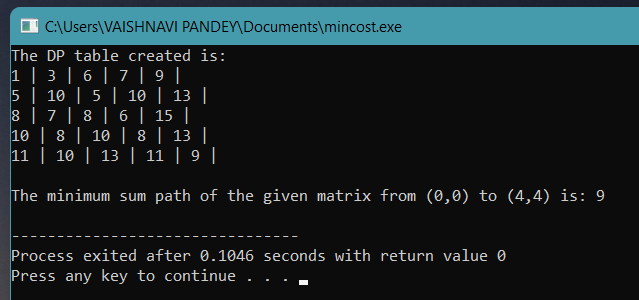#### Java Implementation

``````// Java Implementation

import java.util.*;

public class MinimumSumPath{

static void printCostMatrix(int costMat[][]){

//printing the min cost table
System.out.println("The Updated Cost Matrix is:");
for(int i=0; i<R; i++){
for(int j=0; j<C; j++){
System.out.print(costMat[i][j] + " | ");
}
System.out.println();
}
}

static int R = 5;
static int C = 5;

static int CalculateMinCost(int cost[][])
{

for (int i = 1; i < R; i++)
{
cost[i] += cost[i - 1];
}

for (int j = 1; j < C; j++)
{
cost[j] += cost[j - 1];
}

for (int i = 1; i < R; i++)
{
for (int j = 1; j < C; j++)
{
cost[i][j] += Math.min(cost[i - 1][j - 1],
Math.min(cost[i - 1][j],
cost[i][j - 1]));
}
}

//Printing the cost matrix
printCostMatrix(cost);
//Minimum SUm Path
return cost[R - 1][C - 1];
}

// Driver code
public static void main(String[] args)
{
int costMatrix[][] = {      {1, 2, 3, 1, 2},
{4, 9, 2, 5, 6},
{3, 2, 3, 1, 9},
{2, 1, 3, 2, 7},
{1, 2, 5, 3, 1} };
System.out.print("The minimum sum path of the given matrix from (0,0) to (4,4) is: "+CalculateMinCost(costMatrix) + "\n");

}
}``````

#### Output: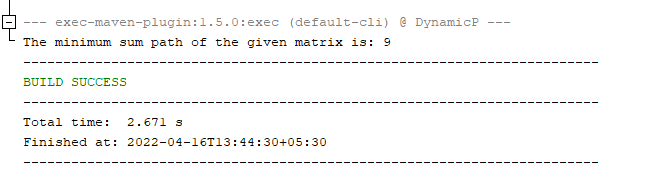#### Python Implementation

``````R, C = 5, 5
def printCostMatrix(costMat):
# printing the min cost table
print("The DP table created is:")
for i in range(R):
for j in range(C):
print(costMat[i][j], "|", end = "")
print("")

def calcMinCost(costMat):

for i in range(1, R):
costMat[i] += costMat[i-1]

for j in range(1, C):
costMat[j] += costMat[j-1]
for i in range(1, R):
for j in range(1, C):
costMat[i][j] += min(costMat[i-1][j-1], min(costMat[i-1][j], costMat[i][j-1]))

printCostMatrix(costMat)

return costMat[R-1][C-1]

def main():
costMat = [ [1, 2, 3, 1, 2], [4, 9, 2, 5, 6], [3, 2, 3, 1, 9 ],[2, 1, 3, 2, 7], [1, 2, 5, 3, 1] ]

res = calcMinCost(costMat)
print("The minimum sum path of the given matrix is: ", res)

main()
``````

#### Output: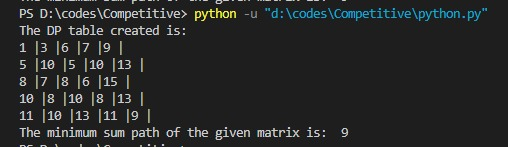#### Points to be noted:

1.  The table contains the minimum sum path from (0,0) to that individual cell.
2. In the given code, we pass the destination coordinates, which is (4,4). Thus the minimum sum path obtained is the minimum sum of the path from (0,0) to (4,4).

## Time and Space Complexity

• Time Complexity
In this approach, we use the concept of dynamic programming. We fill the DP table using recursion by traversing the whole given matrix once. Thus, the time complexity is,

T(n) = O(R*C),

where R is the number of rows and C is the number of columns.

• Space Complexity
In this approach, we create a table DP to store the minimum sum path of our matrix. The dimensions of the DP table are the same as the input matrix, that is, R*C. Thus,

Space Complexity = O(R*C),

where R is the number of rows and C is the number of columns.

This might seem the best solution to some, but in reality, there is still a better solution than this where we make changes directly to the input matrix. This does not affect our time complexity but drastically affects our space complexity.

1. What is an alternative method to solve the minimum sum path in a matrix?
It is possible to solve the minimum sum path in a matrix using Dijkstra’s shortest path algorithm.

To know more about Dijkstra’s algorithm, read this blog: A Guide to Master Graph Algorithms for Competitive Programming

## Conclusion

To summarize, in this article, we discussed the problem statement of the minimum sum path in a matrix, followed by a thorough explanation of the three possible approaches, their codes, and output.